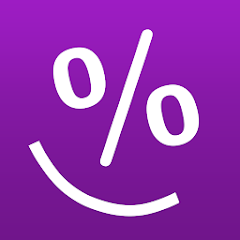# Percentage Calculator

5K+Everyone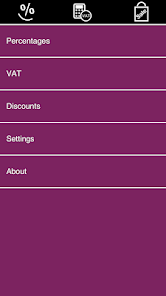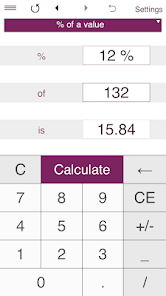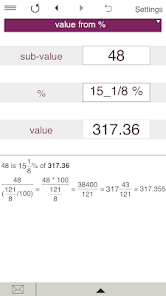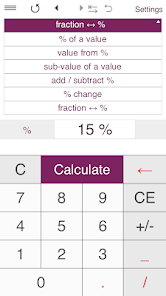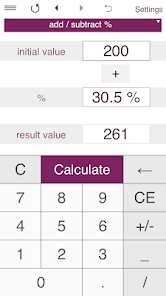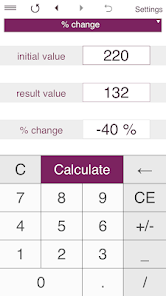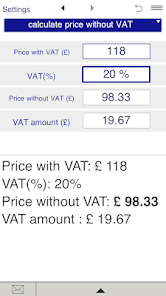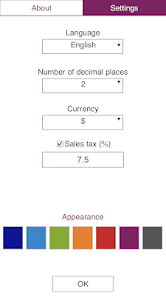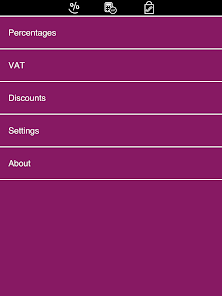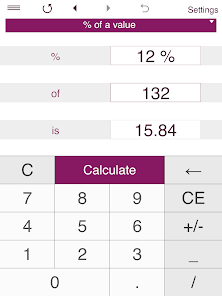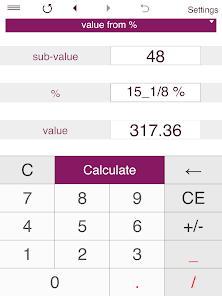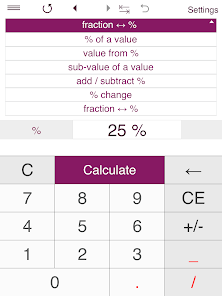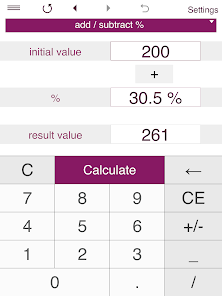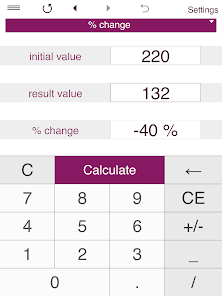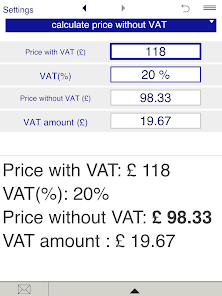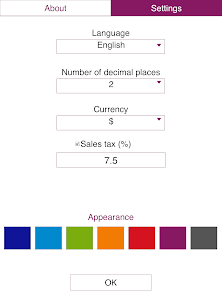Smart Percentage Calculator is a must-have app for everyday calculations - simple percentage calculations, percentage increase/decrease, VAT calculations, calculating tips, sale price, percentage off, discounted price, and more.

FEATURES:
• SIMPLE PERCENTAGE:
- finds the percentage of a value (what is 5% of 20?)
- finds the value from a percentage - finds the whole number when you know the percentage and the sub-value (if 4 is 30%, what is the whole number?)
- sub-value of a value - finds the proportion of one number to another in percentage terms (20 is what percent of 150?)

• ADD OR SUBTRACT A PERCENTAGE

• PERCENTAGE CHANGE
Finds the percentage change (increase/decrease) between two values.

• FRACTION TO PERCENTAGE
Converts fractions to percentages and percentages to fractions.

• VAT
- calculates the price without VAT (net price).
- calculates the price with VAT (gross price).
- calculates the VAT amount.

• DISCOUNT
- calculate the discounted price and your saving
- handles multiple discounts

Smart Percentage Calculator makes percentage calculations based on various inputs including values, sub-values, percentages, ratios, fractions, percentage changes, percentage increase/decrease.
If any two of values are entered, the calculator finds the third one.
For VAT calculations you may enter any VAT rate.
Discount calculations: just enter the original price, discount, sales tax percentage (if applicable) and get the final price and the amount of money you save.You can enter discount percentage or discount amount.

You can select rounding to the specified number of decimal places from 0 to 3. By default, the app displays 2 decimal places.
To round results to the nearest whole number, select 0 decimal places.

Supports fractional values.

You can make calculations in one of 15 supported currencies.

History tape to view recent calculations (25 entries). Back and forward buttons lets you view your recent calculations.

Share results and history via email.

'Undo' for the Clear command.

Supports both portrait and landscape orientation.
Updated on
Jul 30, 2020

## Data safety

Developers can show information here about how their app collects and uses your data. Learn more about data safetyNo information available